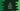# 4 ways in C# to print the Geometric Progression or GP## C# program to print the Geometric Progression series:

The Geometric Progression series is a sequence of non-zero numbers where each value of the series is calculated by multiplying the previous number by a constant number. This constant number is called the common ratio.

For example, if the starting number of the Geometric Progression series is a and common ratio is r, the Geometric Progression series will be a, ar, ar^2, ar^3…etc.

The program we will write will take the values of a, r, and n i.e. the number of values to print as inputs from the user, and it will print the Geometric Progression series up to n terms.

### Method 1: C# program to print the Geometric Progression by using a for loop:

Let’s use a for loop to print the Geometric Progression series in C#. This program will take the start number, common ratio, and the number of items to print as inputs from the user.

``````using System;

namespace dotnetsample
{
class Program
{
static void PrintGP(int a, int n, int r)
{
for (int i = 0; i < n; i++)
{
Console.Write("{0} ", a * Math.Pow(r, i));
}
}
static void Main(string[] args)
{
int a, n, r;

Console.WriteLine("Enter the first number of the G.P.: ");

Console.WriteLine("Enter the total numbers to print: ");

Console.WriteLine("Enter the common ratio: ");

PrintGP(a, n, r);
}
}
}``````

Here,

• Integer variables a, n, and r are used to hold the first number of the G.P., total numbers to print, and common ratio of the series. It asks the user to enter these values, reads the values, and stores these in the variables.
• PrintGP method is used to print the Geometric Progression series. It takes the values of a, n, and r as its parameters and prints the first n numbers of the series.
• In PrintGP, we are using a for loop to print the series. It runs from 0 to n-1. On each iteration, it multiplies the value of a with r^i. Math.Pow method is used to find the power.

If you run this program, it will print the output as below:

``````Enter the first number of the G.P.:
1
Enter the total numbers to print:
10
Enter the common ratio:
3
1 3 9 27 81 243 729 2187 6561 19683``````

### Method 2: C# program by using a for loop and without Math.Pow:

We can also remove the call of Math.Pow and multiply the common ratio with the previous value of the series to get the current value. Let’s write the above program without using Math.Pow:

``````using System;

namespace dotnetsample
{
class Program
{
static void PrintGP(int a, int n, int r)
{
int CurrentValue = a;

for (int i = 0; i < n; i++)
{
Console.Write("{0} ", CurrentValue);
CurrentValue *= r;
}
}
static void Main(string[] args)
{
int a, n, r;

Console.WriteLine("Enter the first number of the G.P.: ");

Console.WriteLine("Enter the total numbers to print: ");

Console.WriteLine("Enter the common ratio: ");

PrintGP(a, n, r);
}
}
}``````
• CurrentValue holds the current value to print. It is equal to a at the start of the series.
• Inside the loop, we are printing this value and updating the CurrentValue by multiplying it by r, which will be the next value of the series.

It will give similar output.

### Method 3: By using a while loop to print the G.P. in C#:

We can replace the for loop with a while loop to get the same result. Let me modify the above program to use a while loop to print the G.P. series:

``````using System;

namespace dotnetsample
{
class Program
{
static void PrintGP(int a, int n, int r)
{
int CurrentValue = a, i = 0;

while (i < n)
{
Console.Write("{0} ", CurrentValue);
CurrentValue *= r;
i++;
}
}
static void Main(string[] args)
{
int a, n, r;

Console.WriteLine("Enter the first number of the G.P.: ");

Console.WriteLine("Enter the total numbers to print: ");

Console.WriteLine("Enter the common ratio: ");

PrintGP(a, n, r);
}
}
}``````

This is almost similar to the above program. The only difference is that we are initializing the initial value of i before the loop starts, and its value is incremented by 1 at the end of each iteration of the while loop. It will print a similar result.

### Method 4: Recursive method to print the G.P. in C#:

Let’s use a recursive method to print the G.P.. A recursive method calls itself again and again and it needs to be stopped based on some specific condition. We can convert the PrintGP method to a recursive method.

``````using System;

namespace dotnetsample
{
class Program
{
static void PrintGP(int CurrentValue, int n, int r)
{
if (n == 0) return;

Console.Write("{0} ", CurrentValue);
PrintGP(CurrentValue * r, n - 1, r);
}
static void Main(string[] args)
{
int a, n, r;

Console.WriteLine("Enter the first number of the G.P.: ");

Console.WriteLine("Enter the total numbers to print: ");

Console.WriteLine("Enter the common ratio: ");

PrintGP(a, n, r);
}
}
}``````

Here,

• If the value of n is equal to 0, it returns. This check will make sure that the recursive call will stop at one point.
• It prints the value of CurrentValue, which is equal to a on the first call of this method. On each recursive call, we are updating its value to the next value of the series i.e. CurrentValue,r, and the value of n is decremented by 1. So, it will stop after n calls.

You will get a similar result.